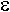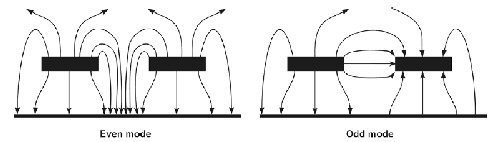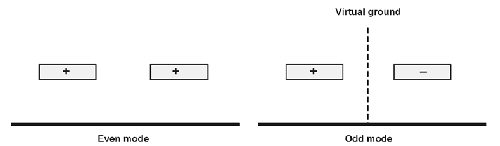# Even and Odd Mode Impedances

This material came from Trev45, who frequents our message boards. To which we say, Thank you sir, may we have another!

In preparing this text, I am acutely aware of being a dwarf climbing onto the shoulders of comparative giants. Much of the credit must go to Polar Instruments Ltd., who produced a very informative Application Note AP157 related to high-speed circuit PCB testing, from which I have shamelessly plagiarized the diagram.

You might ask why bother to explain a topic when the answers can be computed by any of a thousand electromagnetic field simulation software packages?

Well, the short answer is "to understand the answer, you first need to understand the question."

Impedance is a vector quantity that expresses the ratio of electromagnetic voltage (or electric field strength) to current (or magnetic flux density). Imagine the familiar coaxial transmission line, a center circular conductor within a circular ground plane. There is a straightforward analytic formula for the characteristic impedance:

Z0 = (138/ (R)^0.5)* Log(D/d)

where d is the inner diameter, D is the outer diameter, andR the relative permittivity.

Now imagine a simple balanced two-wire line. Again, there is a straightforward analytic formula for the characteristic impedance:

Z0 = (276/ (R)^0.5 )* Log(2*s/ D)

where s is the spacing and D is the conductor diameter, is the relative permittivity

Now imagine a transmission line comprising a balanced twin conductor pair inside a circular conducting tube, in other words a shielded twisted pair transmission cable. Is there a straightforward expression for Z0, and anyway, what is meant by Z0 in this situation? Well, a) there isn't and b) it's complicated. There are three impedances that characterize the structure, characteristic impedance, even mode impedance and odd mode impedance.

Whilst in this case there is no precise analytic formula that applies to any geometry, such as circular-cylindrical or strip-line of any ratio of dimensions, there are approximate formulas that apply to say strip-line or coaxial cylindrical forms over a restricted range of parameters. A reasonably accurate formula for screened twisted pair is to apply a small correction to the balanced line formula to take into account the proximity of the overall screened ground cylinder. The formula is

Z0 = (276/ (R)^0.5) * Log(2*s / D) * ((1 - G2)/ (1 + G2))

G being the ratio of center to center inner conductor spacing to outer diameter (thanks for the correction, Bert!)

There are two modes of current flow in an electromagnetic situation such as this: the first is one flowing down one conductor with a contra-flow current back up the other conductor caused by displacement current coupling between the two conductors. This is termed the odd mode or differential mode current, and has an associated odd mode characteristic impedance, styled Z0o. Imagine it conceptually like this. Energy couples from one line into the other, flowing away from the source to the matched load. No energy returns from the far end matched loads, but some flows back out towards the source, so there is a plus arrow going away down one conductor and a minus arrow coming back out of the other conductor.

The other component of current flows by displacement current between each center conductor carrying the same polarity, and the ground that is common between them. Hence this is called the common or even mode current, and has an associated even mode characteristic impedance, styled Z0e.Figure 1 Even and Odd Mode electric field lines

From the electrostatic field patterns, it is clear that there are 3 values of capacitance involved for the odd mode, and each must figure in the Z0 formula somewhere. The first is the direct capacitance to ground of each trace, the second is the mutual capacitance between the traces, and the third represents the distortion of each caused by the presence of the coupled element (and a small contribution due the thickness of each).

The same argument applies for the inductance when the magnetic fields are considered, and the combined system impedance at very high frequencies in a non-dissipative system is then

Z0 = (L/C)^0.5

where L is the inductance per meter and C is the capacitance per meter.

Whatever the geometry, the following holds true

Z0 = ( Z0o* Z0e)^0.5

which is the characteristic impedance of the system.

Also, intuitively, from an examination of the field pattern, the even mode impedance Z0e is likely to be high because the inductance increases and the capacitance decreases as the coupling becomes tighter. This because the field is more concentrated between the center conductors, and less field exists directed to the common ground

The coupling between the two conductors is put to good use as the well-known transmission component, the directional coupler. The amount of coupled energy resulting from any geometry depends on Z0e and Z0o, or perhaps those two impedances depend on the coupling. Whichever way you view it, they are interrelated and the expressions for ¼ wavelength matched lines are:

Z0e =Z0 * ((1 + k)/(1 - k))^0.5

where k = 10^(-0.05*C) and

C = coupling in decibels, and

Z0 is the line characteristic impedance

Z0o=Z0 * ((1 - k)/(1 + k))^0.5

From these, it is clear that

Z0 = ( Z0o* Z0e )^0.5

In a 50 ohm system, for weak coupling, say 30 dB, k = 0.032, and so Z0e should be around 51.6 ohms and Z0o around 48.42 ohms. Intuitively, the coupling is weak, so the two impedances are almost identical to the system impedance, 50 ohms. With weak coupling there is minimal field distortion relative to the case where only one conductor exists.

For a 3 dB broadside coupler in a 70.7 ohm section (Z0'), k = 0.707 so Z0e is approximately 170.7 ohms and Z0o is 29.3 ohms. Because the coupling is tight, these impedances are considerably different from the system impedance 70.7 ohms.

Trace-widths and spacing in micro-strip or strip-line can be found in the standard reference works, or even some day on this site 8-). To be honest, there are almost as many modeling formulas as there are microwave engineers working in this field.

Or, now you understand the question, fire up your software and you will understand the answer.

Author : Trev45